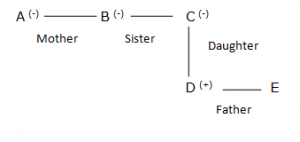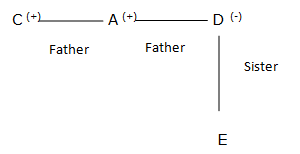Crack IBPS PO : Statements and Assumptions : Reasoning day 57

Direction.1-5) In each question below is given a statement followed by two assumptions number I and II.

An assumption is something supposed or taken for granted. You have to consider the statement and the following assumption and decide which of the assumption Implicit in the statement.

1. Statements: Government has decided to relocate all the factories from the city with immediate effect to reduce pollution.

Assumption:

1. Pollution in the city is being caused only because of the factories existing there.
2. People may be able to manage travelling daily to the relocated factories.

(a) If only assumption I is Implicit.

(b) If only assumption II is Implicit.

(c) If either assumption I or II is Implicit.

(d) If neither assumption I or II is Implicit.

(e) If both assumption I and II are Implicit.

(b) Assumption I is ruled out because of the word only. But II is implicit because without considering this factor relocation won’t make sensr mye.

2. Statements: The college administration has instructed all the students to stop using cell phone with in the college premises.

Assumption:

1. The students to stop using cell phone in the college premises.

II.The Students may be Continue to use cell phone in the college premises.

(a) If only assumption I is Implicit.

(b) If only assumption II is Implicit.

(c) If either assumption I or II is Implicit.

(d) If neither assumption I or II is Implicit.

(e) If both assumption I and II are Implicit.

(a) When you instruct someone to do something you assume that he may do it. Hence II is implicit and II is not.

3. Statements: Many organisations have switched over to online mode of examinations.

Assumption:

1. candidate from all parts of the country may be Well-versed using Computers.
2. Online mode of examinations helps in recruiting more capable personnel.

(a) If only assumption I is Implicit.

(b) If only assumption II is Implicit.

(c) If either assumption I or II is Implicit.

(d) If neither assumption I or II is Implicit.

(e) If both assumption I and II are Implicit.

(a) Assumption I is implicit because only then the switching over makes sense. But II need not be an assumption. The switching over may have been prompted by economic factors or those of convenience.

D.4-5) Use the relationships given and answer the questions that follow

A@B means A is the husband of B

A#B means A is the father of B

A\$B means A is the mother of B

A%B means A is the brother of B

A*B means A is the sister of B

A!B means A is the daughter of B

A&B means A is the son of B

A+B means A is the wife B

4.Which of the following means A is mother of E?

(a) C \$ B * A! D # E

(b) A % B * C \$ D ! E

(c) A # B * C ! D \$ E

(d) A \$ B * C! D # E

(e) None of these5.Which of the following combination comes in the hyphen C Grandfather D and E?

C_A_D_E

(a) *, #, \$

(b) *,#,&

(c) #,#,*

(d) #, %, *

(e) None of theseDirection.6-10) Study the following information carefully and answer the given questions.

When the word and number arrangement machine is given an input line of words and numbers, it arranges them following a particular rule. The following is an illustration of an input and rearrangements

Input: Shift 30 Tender doll 35 28 Words 46 Ice 53 74 Always

Step1: Words 74 shift 30 Tender Doll 35 28 46 Ice 53 Always

Step2: Words 74 Tender 53 shift 30 Doll 35 28 46 Ice Always

Step3: Words 74 Tender 53 shift 46 30 Doll 35 28 Ice Always

Step4: Words 74 Tender 53 shift 46 Doll 35 30 28 Ice Always

Step5: Words 74 Tender 53 shift 46 Doll 35 Always 30 28 Ice

Step6: Words 74 Tender 53 shift 46 Doll 35 Always 30 Ice 28

Step 6 is the last step of the above input.

As per the rules followed in the above steps, find out the appropriate steps for the given input

INPUT: Elephant 55 Inbuilt 89 outer temple 27 75 ugly clear 59 Pink 16 12

Step 1: Temple 89 elephant 55 inbuilt outer 27 75 ugly clear 59 pink 16

Step 2: Temple 89 pink 75 elephant 55 inbuilt outer 27 ugly clear 59 16

Step 3: Temple 89 pink 75 elephant 59 55 inbuilt outer 27 ugly clear 16

Step 4: Temple 89 pink 75 elephant 59 clear 55 inbuilt outer 27 ugly 16

Step 5: Temple 89 pink 75 elephant 59 clear 55 inbuilt 27 outer ugly 16

Step 6: Temple 89 pink 75 clear 59 elephant 55 inbuilt 27 outer ugly 16

6.How many steps will be required to complete the given arrangements?

(a) Six

(b) Seven

(c) Five

(d) Eight

(e) None of these

(c) Five

7.If the last step all the words get rearranged in alphabetical order, which of the following word will remain as its Original Position?

(a) Inbuilt

(b) Ugly

(c) Pink

(d) Temple

(e) None of these

(b) Ugly

8.Which of the following would be at the fifth position from the left in step5?

(a) Inbuilt

(b) Clear

(c) 59

(d) Elephant

(e) None of these

(b) Clear

9.Which step number would be the following Output?

“Temple 89 Pink 75 Clear 59 Elephant 55 Inbuilt Outer 27 Ugly 16 12”

(a) Step 3

(b) Step 2

(c) Step 5

(d) Step 4

(e) None of these

(a) Step 3

10.How many words/numbers and there between ‘89’ and ‘Inbuilt’ in step 3?

(a) 6

(b) 4

(c) 5

(d) 2

(e) None of these